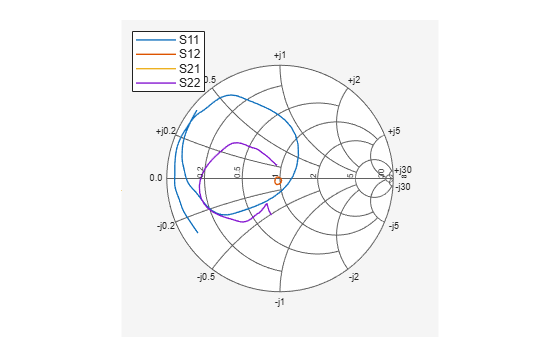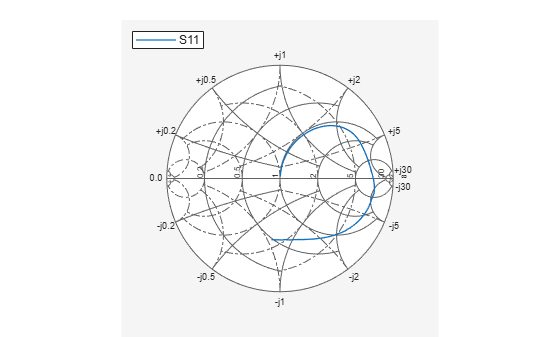# smithplot

Plot measurement data on Smith chart

## Syntax

``smithplot(data)``
``smithplot(frequency,data)``
``smithplot(ax,___)``
``smithplot(hnet)``
``smithplot(hnet,i,j)``
``smithplot(hnet,[i1,j1;i2,j2;....,in,jn])``
``smithplot(rfbudgetobj,i,i)``
``s = smithplot(___)``
``s = smithplot('gco')``
``smithplot(___,Name,Value)``

## Description

````smithplot(data)` creates a Smith chart based on input data values. NoteThe Smith chart is commonly used to display the relationship between a reflection coefficient, typically given as S11 or S22, and a normalized impedance. ```
````smithplot(frequency,data)` creates a Smith chart based on frequency and data values.```
````smithplot(ax,___)` creates a Smith chart with a user defined axes handle, `ax`, instead of the current axes handle. Axes handles are not supported for network parameter objects. This parameter can be used with either of the two previous syntaxes.```

example

````smithplot(hnet)` plots all the network parameter objects in `hnet`.```

example

````smithplot(hnet,i,j)` plots the (i,j)th parameter of `hnet`. `hnet` can be a network parameter, an rfckt, an rfdata,or an nport object.```
````smithplot(hnet,[i1,j1;i2,j2;....,in,jn])` plots multiple parameters (i1, j1, i2, j2, …, in, jn) of `hnet`. `hnet` can be a network parameter, an rfckt, an rfdata, or an nport object.```
````smithplot(rfbudgetobj,i,i)` plots the reflection coefficient of an `rfbudget` object. NoteFor rfbudget objects, smith plot is restricted to reflection coefficients. ```
````s = smithplot(___)` returns a Smith chart object handle so you can customize the plot and add measurements. ```
````s = smithplot('gco')` returns a Smith chart object handle of the current plot. This syntax is useful when the function handle, `p` was not returned or retained.```
````smithplot(___,Name,Value)` creates a Smith chart with additional properties specified by one or more name-value pair arguments. `Name` is the property name and `Value` is the corresponding property value. You can specify several name-value pair arguments in any order as `Name1`, `Value1`, `...`, `NameN`, `ValueN`. Properties not specified retain their default values.For list of properties, see SmithPlot Properties. NoteThe property `'Parent'` might be used to control the location where Smith chart gets plotted. ```

## Examples

collapse all

Use the Smith plot interactive menu for changing line and marker styles.

Plot the Smith plot of s-parameters data file, `default.s2p`.

`data = nport('default.s2p')`
```data = nport: N-port element NetworkData: [1x1 sparameters] Name: 'Sparams' NumPorts: 2 Terminals: {'p1+' 'p2+' 'p1-' 'p2-'} ```
`smithplot(data)`Right click on the S11 line to reveal interactive menu, `DATASET 1. `Use LineStyle to change the style of S11 line on the Smith plot.Plot the Smith plot of S11 of s-parameter data file using an impedance of 75 ohms.

```data = sparameters('passive.s2p' ); s = sparameters(data,75); p = smithplot(s,1,1, 'GridType','ZY');```Use the data cursor icon in the toolbar to insert a cursor on your smith plot chart. You now know the S11, VSWR, Impedance, and frequency values at that cursor. For admittance value, change the Grid Type.Plot the Smith chart of an rfckt.amplifier object.

```S = read(rfckt.amplifier,'passive.s2p'); ports = [1,1;1,2]; s = smithplot(S,ports); s.LineStyle = {'--', '-'}; ```This example shows how to plot impedance data on a smithplot

Define impedance data

```z1 = 0.1*50 + 1j*(0:2:50); z2 = (0:2:50) - 0.6*50j;```

Characteristic Impedance

`z0 = 50;`

Convert impedance data to reflection coefficient

```gamma1 = z2gamma(z1,z0); gamma2 = z2gamma(z2,z0);```

Plot the impedance data on the smithplot

```s = smithplot(gamma1,'Color',[0.2 0 1],'GridType',"Z"); hold on; s = smithplot(gamma2,'Color','g','LineStyle','-.','LineWidth',1); s.Marker = {'+','s'}``````s = smithplot with properties: Data: {[26x1 double] [26x1 double]} Frequency: {[] []} Show all properties, methods ```

## Input Arguments

collapse all

Input data, specified as a complex vector or complex matrix.

For a matrix D, the columns of D are independent data sets. For N-by-D arrays, dimensions 2 and greater are independent data sets.

Data Types: `double`
Complex Number Support: Yes

Frequency data, specified as a real vector.

Data Types: `double`

Input objects, specified as one of the following:

• RF Toolbox™ network parameter object

• rfckt object

• rfdata object

• nport object

Data Types: `object`

Input object, specified as

• rfbudget object

Data Types: `object`

## Output Arguments

collapse all

Smith chart object handle. You can use the handle to customize the plot and add measurements using MATLAB® commands.

## Tips

• To list all the property `Name,Value` pairs in `smithplot`, use `details(s)`. You can use the properties to extract any data from the Smith chart. For example, `s = smithplot(data,'GridType','Z')` displays the impedance data grid from the Smith chart.

• For a list of properties of `smithplot`, see SmithPlot Properties.

• You can use the `smithplot` interactive menu to change the line and marker styles.